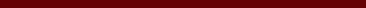### BEBERAPA KELAS GRAF RAMSEY MINIMAL UNTUK LINTASAN P_3 VERSUS P_5

#### Abstract

In 1930, Frank Plumpton Ramsey has introduced Ramsey's theory, in his paper titled On a Problem of Formal Logic. This study became morepopular since Erdős and Szekeres applied Ramsey's theory to graph theory. Suppose given the graph F, G and H. The notation F → (G, H)  states thatfor any red-blue coloring of the edges of F implies F containing a red subgraph of G or a blue subgraph of H. The graph F is said to be the Ramsey graph for graph G versus H (pair G and H) if F → (G, H). Graph F is called Ramsey minimal graph for G versus H if  first, F → (G, H) and second, F satisfies the minimality property i.e. for each e ∈ E (F), then F-e ↛ (G, H). The class of all Ramsey (G, H) minimal graphs is denoted by (G, H). The class (G, H) is called Ramsey infinite or finite if  (G, H) is infinite or finite, respectively. The study about Ramsey minimal graph is still continuously being developed and examined, although in general it is not easy to characterize or determine the graphs included in the (G, H), especially if  (G, H) is an infinite Ramsey class. The characterization of graphs in (, ) has been obtained. However, the characterization of graphs in (, ), for every 3 ≤ m < n is still open. In this article, we will determine some infinite classes of Ramsey minimal graphs  for paths  versus .

#### Full Text:

FULL PDF

DOI: http://dx.doi.org/10.17977/um055v2i12021p14-18

### Refbacks

• There are currently no refbacks.